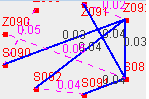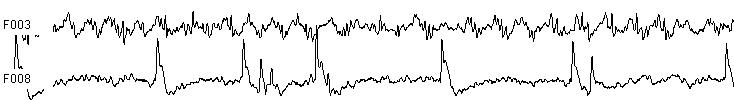Brain Graph Youcan

# Demo for FWHVA

Mapping a time series into a graph

Reference:

• Guohun Zhu, Yan Li, Peng (Paul) Wen, Epileptic seizure detection in EEGs signals using a fast weighted horizontal visibility algorithm, Computer Methods and Programs in Biomedicine, Available online 15 April 2014, ISSN 0169-2607, . online version

## Demo for a sine wave and return the mean degree, mean strength FWHVA

```t=0:.1:pi*10;
t1=0:.03:pi*3;
t2=0:.07:pi*7;
y=sin(t)+cos(t1)+sin(t1);
ouput1=FWHVA(y);
disp('mean degree')
disp(ouput1(1))
disp('mean Strength')
disp(ouput1(2))
disp('mean Repeat')
disp(ouput1(4))
```
```mean degree
3.6317

mean Strength
3.6317

mean Repeat
2.2921

```

## Demo for sine wave and it was inputed int a degree sequence FWHVA

```output=FWHVA(y,'D');
figure;
subplot(2,1,1)
plot(t,y,'Color',[0,0.7,0.9])
title('Plot for input signals')
xlabel('time points')
ylabel('amplify')

subplot(2,1,2)
plot(t,output,'Color',[0,0.7,0.9])
title('Plot for Degree sequence')
xlabel('time points')
ylabel('degree of HVG')
```